• No products in the cart.

# ATOMIC NUCLEUS A-LEVEL PHYSICS

##### This Unit is about Atomic Nuclei and the theories involved in it.

Atomic Nucleus

Rutherford’s model of the atom

All the positive charge of the atom is concentrated in a small region called the nucleus of diameter less than 10-10m. The negative charge surrounds the positive charge.

This was verified by Rutherfold and his team. The experiment involved the scattering of thin Gold foil.

Alpha particles emitted by a radioactive source were directed towards a thin gold foil. The scattered alpha particles were observed on a fluorescent screen on the focal plane of the microscope. Scintillations were observed on the screen whenever the alpha particles struck the ZnS scintillation detector. The microscope was moved to different positions in order to detect the alpha particles.

Observations

1. The majority of the alpha particles passed through undeflected.
2. A few of the alpha particles were scattered through small angles.
3. Very few alpha particles were deflected through angles greater than 90º.Conclusion

1. The alpha particles being positively charged, their scattering must be due to the positive charge in the gold atom.
2. Since the majority of the alpha particles passed through undeflected, most of the space inside the atom is empty.
3. Large angle scattering occurred whenever an alpha particle was incident almost head on to the nucleus.
4. Since very few alpha particles were scattered through large angles, it follows that the probability of a head on collision with the nucleus is small and it follows that the nucleus occupies only small proportion of the available space inside an atom.

THIS VIDEO IS ABOUT ATOMIC NUCLEUS IN PHYSICS

Closest distance of approach of alpha particles.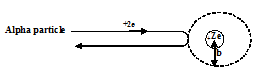Where Z is the proton number or atomic number of the atom.

At closest distance of approach , all alpha particle’s kinetic energy is converted into electrostatic potential energy of the alpha particle or nucleus system.

Hence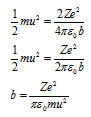Example

A beam of alpha particles of energy 4.2MeV is incident normal to a gold foil. What is the closest distance of approach by the particles to the nucleus of the gold atom?

( Atomic number of gold = 79)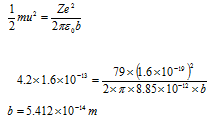Summary, the atom consists of the following main particle: (i) the protons which are positively charged, (ii) the neutrons which carry no charge and the electrons which are found in orbits around the nucleus. The neutrons and protons make up the nucleus of the atom.

THIS VIDEO EXPLAINS MORE ABOUT Rutherford’s model of the atom

The Bohr model of the atom

Bohr postulated that:

• Electrons in atoms can exist only in certain discrete orbits and while in these orbits, they don’t radiate energy.
• Whenever an electron makes a transition from one orbit to another of lower energy, a quantum of electromagnetic radiation is given off.

The energy of the quantum of radiation emitted is given by , where Ei is energy of the electron in the initial orbit, Ef is the energy of the electron in the final orbit, h is Planck’s constant and f is the frequency of emitted electron.

The angular momentum of an electron in its orbit in an atom is an integral multiple ofThe orbit with the lowest energy is called the ground state. All physical systems are in physical equilibrium in the lowest energy state. Other high energy levels are called excited state.

Bohr’s theory of the hydrogen atomNote: (i) The energy of the electron is always negative. This means that work has to be done to move the electron to infinity where it is considered to have zero energy. The electron is therefore bound to the nucleus.

(ii) Whenever an electron makes a transition from a higher energy level, ni, to a lower energy level, nf, the energy of the quantum of radiation emitted is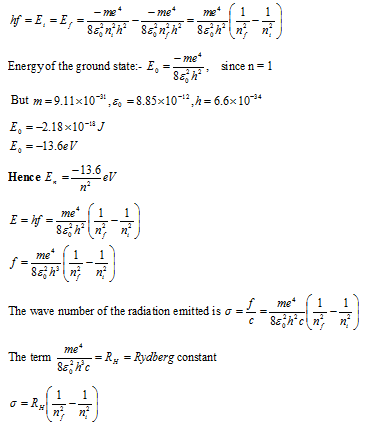Spectral lines of hydrogen atoms

Energy levels are grouped into shells. Electrons in one shell have nearly the same energy. The shells are denoted by letters K, L, M, N etc. where K corresponds to n = 1, L to n = 2, M to n = 3 and so on.

Transitions of electron from a high energy level to lower energy level cause electron to lose energy hence producing electromagnetic waves. Transitions from other shells to K- shell emit spectra of wavelength grouped into what is called Lymann series.

Lymann series lie in the Ultra violet region of the spectrometer.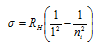Where ni = 2, 3, 4…….

Transitions from other high energy levels to the L- shell ( n = 2), emits spectra of wavelengths referred to as Balmer series. Balmer series lie in the visible spectrum.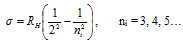Transition from other high energy levels to the M – shell ( n = 3 ), emits spectra referred to as Paschen series which lie in the infra red region.Note Bohr’s theory is to simple to explain spectra of more complicated atoms however, the following remain valid:

• electrons exist outside the atomic nucleus
• existence of energy levels.
• Emission and absorption of radiation occur in discrete amounts called quanta.

Line emission spectra

When atoms like H2, neon etc. are excited due to some form of heat from a frame or electricity, electron transition may occur to higher energy levels. This makes the atom unstable since energy has increased. Electron transition may occur to a vacancy left  in the lower energy level and radiation  of a definite wavelength or frequency is emitted. A line appear bright against a dark background. The lines are separated which give evidence that energy levels of the atoms are separate.

Line absorption spectra.

An atom’s energy can change by only discrete amounts. If a photon of energy, hf, is just enough to excite the atom, such that an electron can jump to one of higher energy levels, the photon will be absorbed. The intensity of the incident radiation is reduced since it has lost a photon. A dark line on a white background is observed, whose wavelength is that of the absorbed photon.

THIS VIDEO IS ABOUT THE BOHR MODEL OF THE ATOM

Yaaka Digital Network ©, a Ultimate Multimedia Consult product